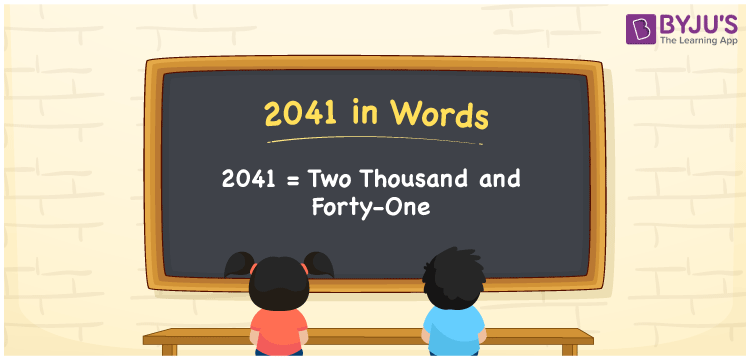# 2041 in Words

2041 in words is written as Two thousand forty-one. In both the International System of Numerals and the Indian System of Numerals, 2041 is written as Two thousand forty-one. The number 2041 is a Cardinal Number as it could represent some quantity. For example, “the speakers cost 2041 rupees”.

 2041 in Words Two thousand forty-one Two thousand forty-one in Number 2041

## 2041 in English Words

2041 in English words is read as “Two thousand forty-one”.## How to Write 2041 in Words?

To write 2041 in words, we shall use the place value chart. In the place value chart, put 2 in the thousands, 0 in the hundreds, 4 in the tens, and 1 in the ones, respectively. Let us make a place value chart to write the number 2041 in words.

 Thousands Hundreds Tens Ones 2 0 4 1

Thus, we can write the expanded form as

2 × Thousand + 0 × Hundred + 4 × Ten + 1 × One

= 2 × 1000 + 0 × 100 + 4 × 10 + 1 × 1

= 2000 + 0 + 40 + 1

= 2041

= Two thousand forty-one.

2041 is a natural number, the successor of 2040 and the predecessor of 2042.

2041 in words – Two thousand forty-one

• Is 2041 an odd number? – Yes
• Is 2041 an even number? – No
• Is 2041 a perfect square number? – No
• Is 2041 a perfect cube number? – No
• Is 2041 a prime number? – No
• Is 2041 a composite number? – Yes

## Frequently Asked Questions on 2041 in Words

Q1

### How to write 2041 in words?

2041 in words is written as Two thousand forty-one.
Q2

### How to write 2041 in the International and Indian System of Numerals?

In both, the system of numerals, 2041 in words, is written as Two thousand forty-one.
Q3

### How to write 2041 in a place value chart?

In the place value chart, write 2 in the thousands, 0 in the hundreds, 4 in the tens, and 1 in the ones, respectively.